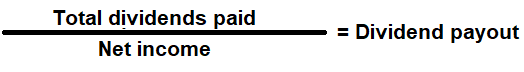# Dividend Payout Ratio Calculator

Key in the Total Dividends and Net Income Values to the respective fields given below and then click Calculate to get the desired result.

# Dividend Payout Ratio Calculator - Glossary:

Dividend Payout Ratio: It is the dollar amount of dividends a company pays out, relative to its total net income.

### Formula:### How to use this equation?

The values are commonly stated against Net Income and total dividends in the income statement. To use this ratio, simply divide total dividends by net income.

Total Dividends:
Cash distributions that are paid over a period of time by the company to the number of outstanding ordinary shares issued.

Net Income:
Profit before deducting all its interest & taxes.

Example:
A company reported a net income of \$100,000 for the year. In the same period, it issued \$30,000 of dividends to its shareholders. Then its dividend payout ratio is 30%. It means it has retained 70% of its net income for reinvestment and paid out 30% of its net income to its ordinary shareholders in the form of dividends.